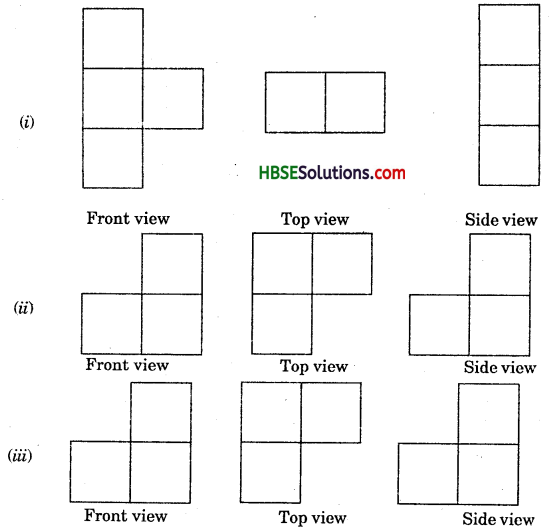# HBSE 7th Class Maths Solutions Chapter 15 Visualising Solid Shapes InText Questions

Haryana State Board HBSE 7th Class Maths Solutions Chapter 15 Visualising Solid Shapes InText Questions and Answers.

## Haryana Board 7th Class Maths Solutions Chapter 15 Visualising Solid Shapes InText Questions

Try These (Page 277)

Question 1.
Match the shape with the name: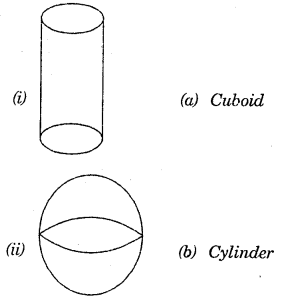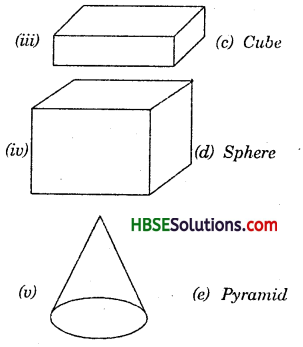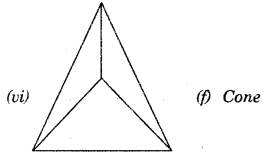Solution:
(i) (b) Cylinder
(ii) (d) Sphere
(iii) (a) Cuboid
(iv) (c) Cube
(v) (f) Cone
(vi) (e) Pyramid

Try These (Page 278)

Question 1.
Match the 2 dimensional figure with the names: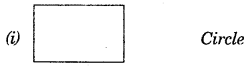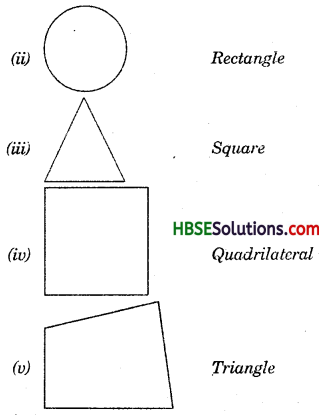Solution:
(i) Rectangle
(ii) Circle
(iii) Triangle
(iv) SquareTry These (Page 279)

Question 1.
Do this : Complete the following table :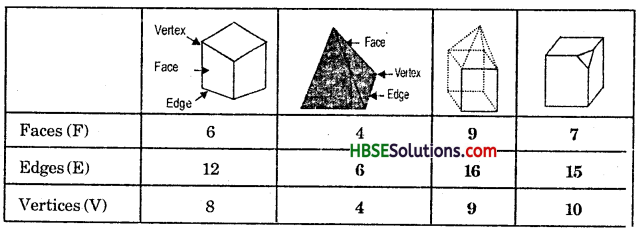Try These (Page 281)

Question 1.
Here you find four nets. There are two correct nets among them to make a tetrahedron. See if you can work out which nets will make a tetrahedron.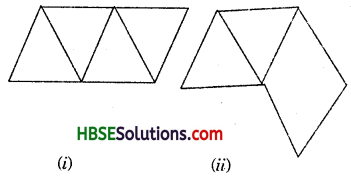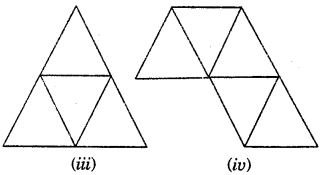Solution: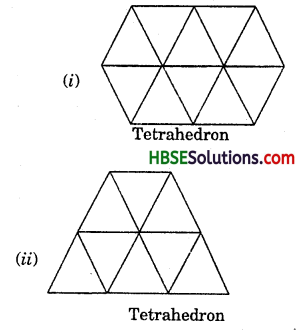Try These (Page 286)

Question 1.
Try to guess the number of cubes in the following arrangements (Fig.):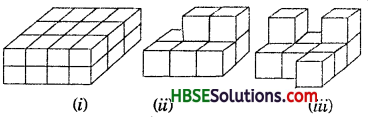Solution:
(i) Numerofcubes = 24
Let the edge of cube = 2 cm
Height = 4 cm
Length = 8cm
(ii) Number of cubes = 8
Let the edge of cube = 2 cm
Length = 6 cm
Height = 4cm
(iii) Number of cubes = 9
Lettheedgeofcube = 2cm
Length = 6cm
Height = 4 cm

Try These (Page 287)

Question 1.
Two dice are placed side by side as shown; Can you say what the total would be on the face opposite to
(а) 5 + 6
(b) 4 + 3
(Remember that in a dice sum of numbers on opposite faces is 7)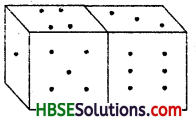Solution:
(i) (a) 5 + 6 → The face opposite to 5 is 2 and 6 is 1.
So, 2 + 1 = 3
(b) 4 + 3 → The face opposite to 4 is 3 and 3 is 4.
So, 3 + 4 = 7.Question 2.
Three cubes each with 2 cm edge are placed side by side to form a cuboid. Try to make an oblique sketch and say what could be its length, breadth and height.
Sol. (Fig.)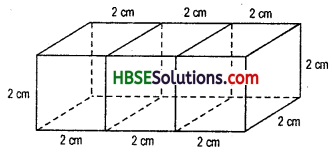Length = 6 cm
Height = 2 cm.

Try These (Page 291)

Question 1.
For each solid, the three views (1), (2), (3) are given. Identify for each solid the corresponding top, front and side views.
Solid In views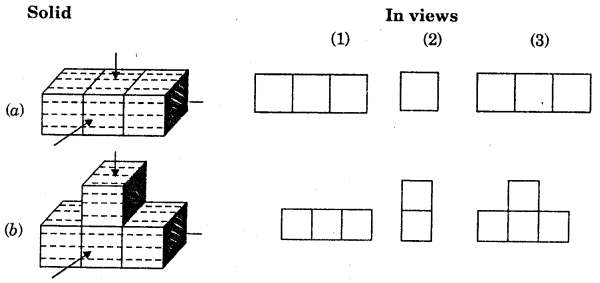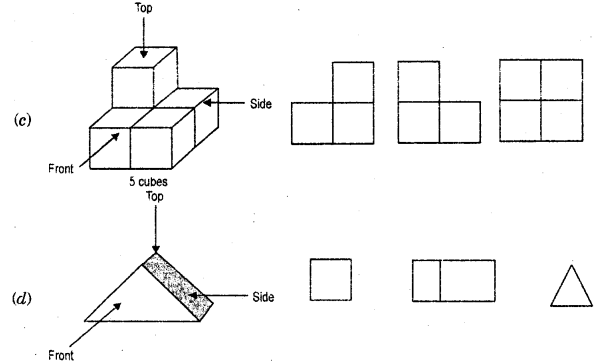Solution: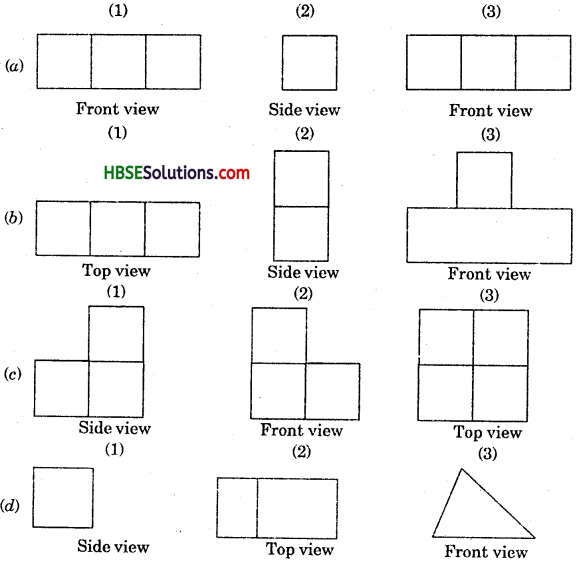Question 2.
Draw a view of each solid as seen from the direction indicated by the arrow.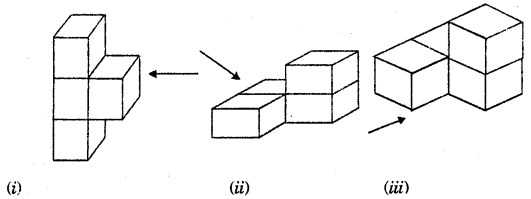Solution: